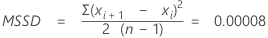# Estimate process variation with individuals data

## Moving range

### What is a moving range?

The moving range measures how variation changes over time when data are collected as individual measurements instead of subgroups. It equals the range of two or more consecutive observations.

### When should I use a moving range?

When data are collected as individual observations, you cannot calculate the standard deviation for each subgroup. In such cases, the average moving range and median moving range across all subgroups are alternative ways to estimate process variation. You can create a control chart of moving ranges to track process variation when you have individual observations.

### Example of the moving range calculations

A department store records the number of seconds it takes for operators to respond to customer calls. For six consecutive calls, the response times are: 22, 35, 40, 20, 10, and 15. To calculate a moving range of length 2, use the absolute value of the difference between two consecutive data points.
Response time Range of values Moving range, with length 2
22
35 (35−22) 13
40 (40−35) 5
20 (20−40) 20
10 (10−20) 10
15 (15−10) 5

You may want to use moving ranges of different lengths if the data are cyclical. For example, if you collect quarterly data, you might use a moving range of length 4 to ensure that one observation from each season is included in the calculation. To do this, subtract the minimum value from the maximum value of four consecutive observations. If you want to calculate a moving range of length 4 for the above example, the first moving range value is 40 – 20 = 20.

## MSSD

### What is MSSD?

The mean of the squared successive differences (MSSD) is used as an estimate of variance. It is calculated by taking the sum of the differences between consecutive observations squared, then taking the mean of that sum and dividing by two.

Two common applications are:
• Basic Statistics - A common application for the MSSD is a test to determine whether a sequence of observations is random. In this test, the estimated population variance is compared with MSSD.
• Control Charts - MSSD can also be used to estimate the variance for control charts when the subgroup size is 1.

### When to use MSSD to estimate standard deviation

For cases when you can't assume that two successive points form a rational subgroup and use the moving range methods, the MSSD method provides an alternative. To use as an estimate of standard deviation, take the square root of MSSD.

### Example of calculating MSSD

For example, suppose you are collecting data on a machine that fills vials of vaccine. You want to make sure the machine dispenses randomly, that is without any special cause of variation. The fill volumes of 12 vials are:
 0.500 ml 0.480 ml 0.490 ml 0.500 ml 0.505 ml 0.500 ml 0.490 ml 0.498 ml 0.500 ml 0.479 ml 0.490 ml 0.510 ml
1. To calculate MSSD, subtract 0.480 ml from 0.500 ml for the first difference. The difference is 0.02.
2. Subtract 0.490 ml from 0.480 ml for the second difference. The difference is −0.01.
3. Continue until you have 11 differences.
4. Divide this sum by 22, or 2 times (n−1).By using this site you agree to the use of cookies for analytics and personalized content.  Read our policy